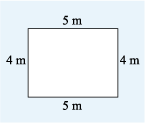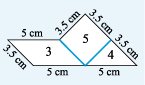Science, Maths & Technology

### Become an OU studentSucceed with maths: part 2

Start this free course now. Just create an account and sign in. Enrol and complete the course for a free statement of participation or digital badge if available.

# 2 Perimeters

One of the properties of a shape that is measured is its perimeter. This is the distance around the edge of a shape. For a shape with straight edges, you can work out the perimeter by measuring the length of each edge and then adding these together.

For example, one practical application of this would be someone wanting to decorate the room shown in Figure 12, using a border along the top of all the walls. To determine the length of the border they would need to work out the perimeter. So the border would measure:

An alternative way to calculate this would be to note that the pairs of sides are the same length, giving us:Figure 12 What is the perimeter of this rectangle?

Have a go yourself in this next activity.

## Activity 2 Around the edge

Timing: Allow approximately 5 minutes
• a.This figure is formed from three of the shapes. What is its perimeter?Figure 13 Figure formed from three of the shapes